# m500 cannot hovering with VIO algorithm

• Hello, after I reset the VOXL m500 to the factory setup, i ran to take-off in the position mode but the quality of VIO algorithm is quite bad, the M500 take off then drift so much and cannot hovering, I had tested so many time but the result was still remained.

I was confirmed the data from local position is quite good by voxl-vision-px4 --debug_odometry. Then I go ahead to check the parameter of EKF2_, there is nothing strange. The radio signal is still good. what's more, in the base floor I used the a big chess board plate for enhance the UAV Vision algorithm when takeoff but it's not work.
We have attached UAV in a test frame so UAV cannot be damaged in some emergency case.
How can i solve this issue?
Thank you

• Hi @Kaiba-Kun ,

Can you perhaps attach a photo of the test frame?

We do some specific tuning for the frame and perhaps vibration is being introduced that could cause some nasty effects?

Thanks!
Travis

•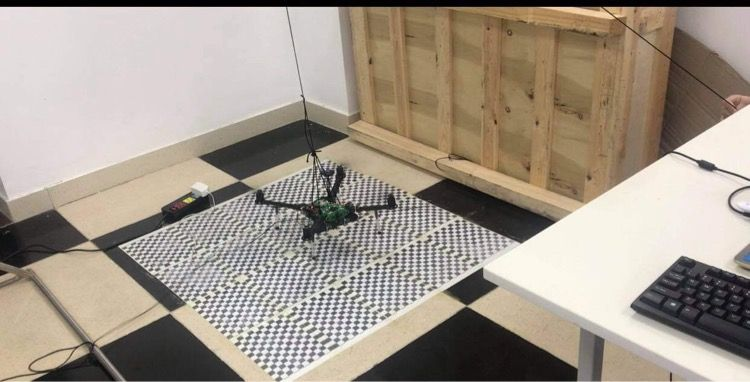Hi. This is the base floor of us. Pls take a look.

• @modaltb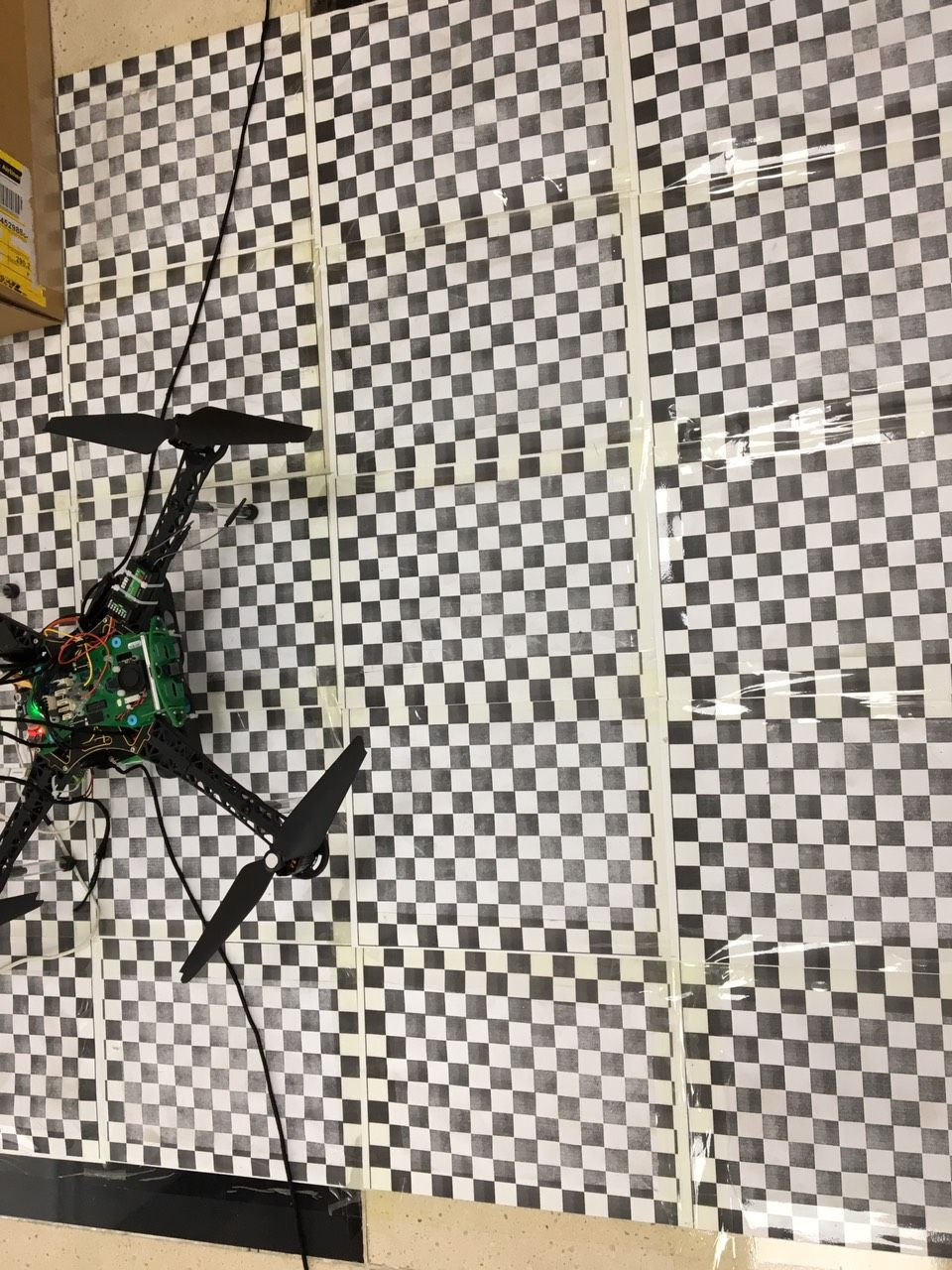•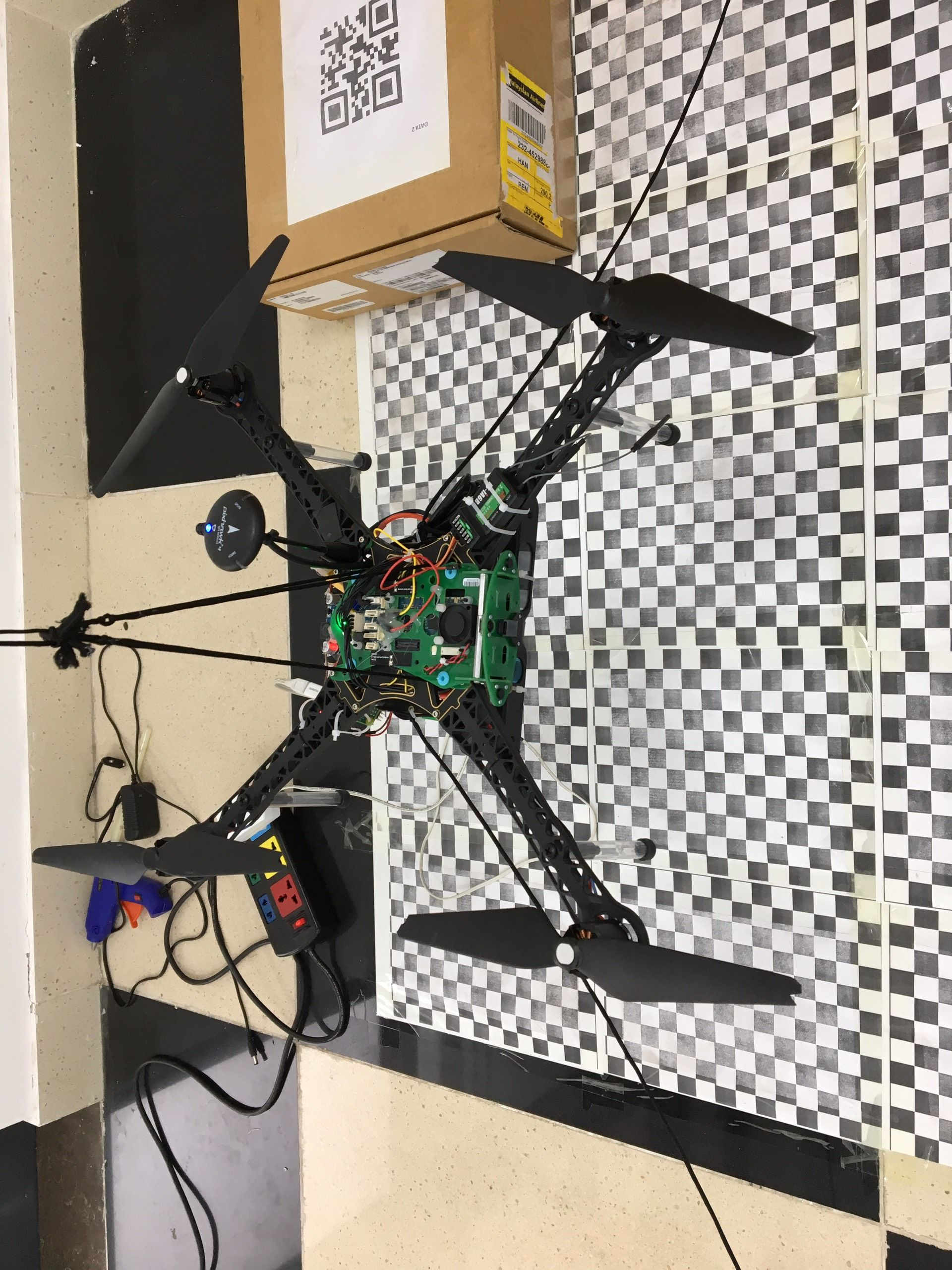and here.

• actually the test frame is only attach in left and right side of UAV. The purpose of test frame is limits UAV in an area so we can avoid the damage when UAV loss control.
@modaltb do you have any idea?

• The system was tested in VIO mode for 20 minutes before shipping. All drones are very sensitive to frame modifications, so this needs to be done carefully

• Were you able to fly successfully before frame modifications?
• How well does it fly manually? Have you looked at the logs in manual.mode for vibration?
• Can you please share a log of the VIO output?
• If you reset all of the software, you may need to recalibrate the cameras
• It looks like there are loose parts hanging off of the drone. VIO is VERY sensitive to vibration. Can you revert your frame modifications and try again?

@Chad-Sweet when the first time our test flight with M500 everything is work properly. you can take a look in this link by the video named before reset.
here

• But after I reset the drone into the factory set up. It's seem the VIO is work with many vibrations, some time it be acceptable.
• In the link above I was captured 3 videos and log file of each, for testing manual with VIO.
• Do I needed to recalibration the camera? I see the data from VIO is quite good when we move the UAV by hand.
• We were revert the UAV to the original state after that but the result is same.

• Also,

• We never try to flight in the manual mode, only use the position and offboard mode.
• I don't think the external material was the reason for this issue because we had attached the component in the bottom of the frame closed as much as possible.
Thank you.

• A couple of ideas:

1. Try to calibrate the tracking camera. Instructions here: https://docs.modalai.com/calibrate-cameras/#calibrate-tracking-camera
2. Insert some foam, preferably memory foam, in between the "green tray" and the frame. This should help reduce vibration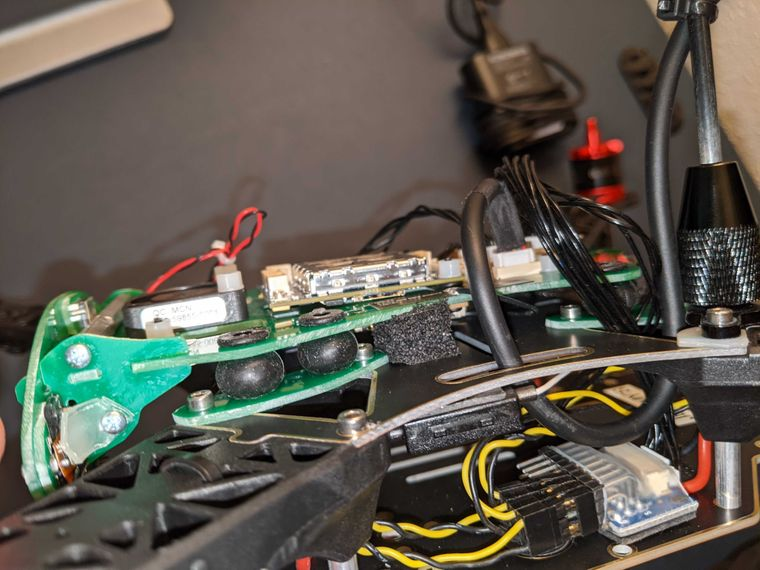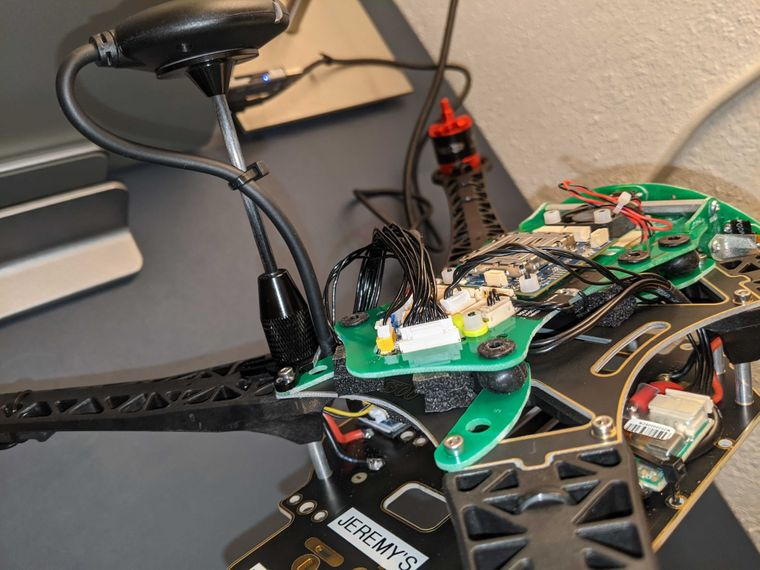I'll try it in the tomorrow. We're in middle of night (GMT +7).

Best regard.

• Can you please send the serial number of the VOXL Flight PCB? We might be able to look up the calibration files in our production database?

• Hi @Chad-Sweet here is the serial number.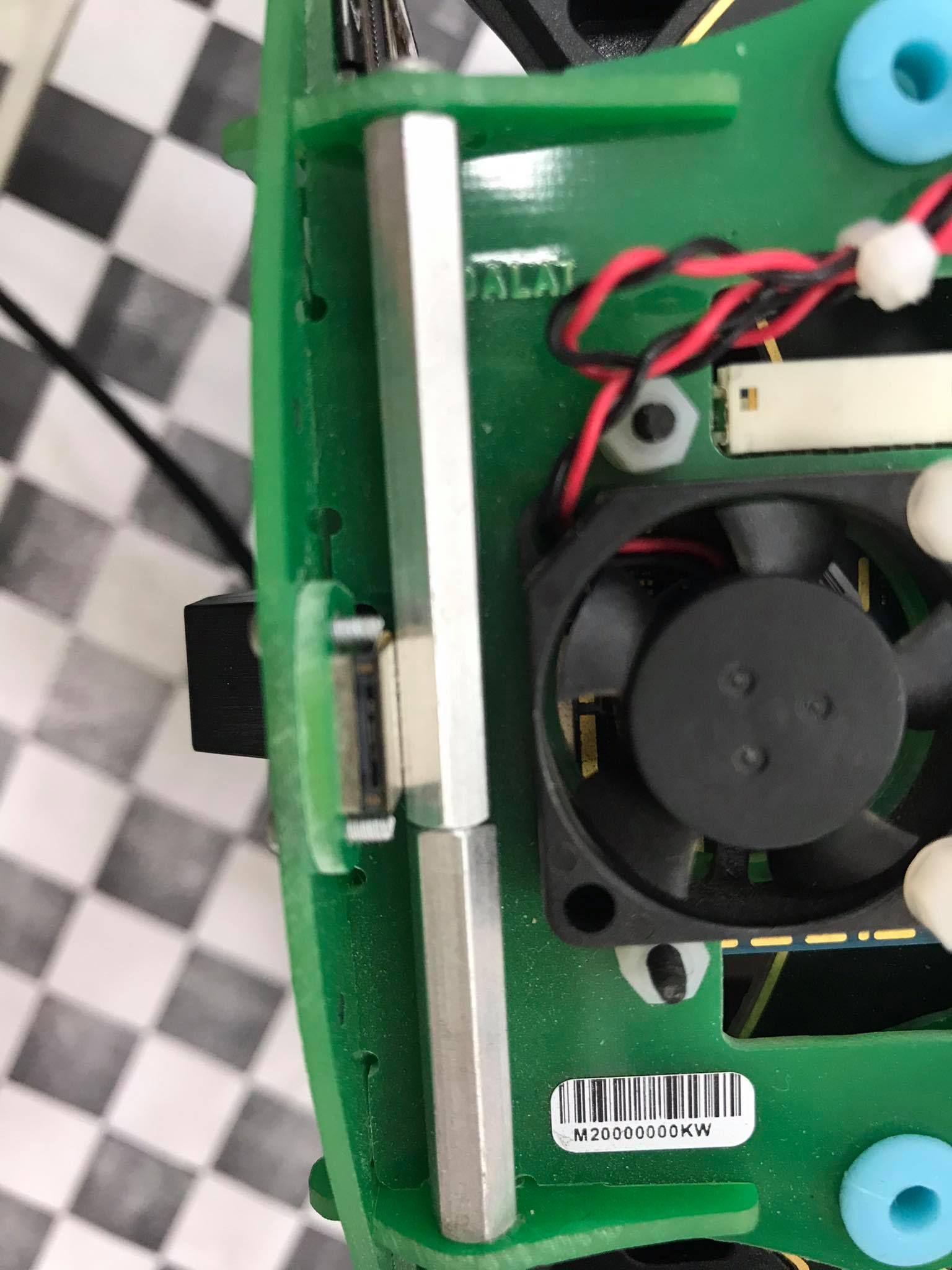• Here's the test data:

Stereo Camera Calibration

left_file:
image_width: 640 image_height: 480 camera_name: stereo/left camera_matrix: rows: 3 cols: 3 data: [492.469682, 0.000000, 287.720563, 0.000000, 492.799801, 241.856917, 0.000000, 0.000000, 1.000000] distortion_model: plumb_bob distortion_coefficients: rows: 1 cols: 5 data: [-0.171887, 0.054112, 0.000816, 0.000653, 0.000000] rectification_matrix: rows: 3 cols: 3 data: [0.999125, 0.024029, 0.034224, -0.023993, 0.999711, -0.001454, -0.034249, 0.000631, 0.999413] projection_matrix: rows: 3 cols: 4 data: [509.343373, 0.000000, 291.779423, 0.000000, 0.000000, 509.343373, 233.497673, 0.000000, 0.000000, 0.000000, 1.000000, 0.000000]

right_file:
image_width: 640 image_height: 480 camera_name: stereo/right camera_matrix: rows: 3 cols: 3 data: [494.055474, 0.000000, 337.960482, 0.000000, 494.170184, 223.591690, 0.000000, 0.000000, 1.000000] distortion_model: plumb_bob distortion_coefficients: rows: 1 cols: 5 data: [-0.182939, 0.076209, 0.000049, 0.002344, 0.000000] rectification_matrix: rows: 3 cols: 3 data: [0.999497, 0.010498, 0.029940, -0.010529, 0.999944, 0.000885, -0.029929, -0.001200, 0.999551] projection_matrix: rows: 3 cols: 4 data: [509.343373, 0.000000, 291.779423, -40.695510, 0.000000, 509.343373, 233.497673, 0.000000, 0.000000, 0.000000, 1.000000, 0.000000]

snav_file:
<SnavCalibration> <SnavCalibrationStereo> <SnavCalibrationLeftStereo> <param name="calib_pixel_width" value="640" /> <param name="calib_pixel_height" value="480" /> <param name="calib_memory_stride" value="640" /> <param name="principal_point_x" value="287.720563" /> <param name="principal_point_y" value="241.856917" /> <param name="focal_length_x" value="492.469682" /> <param name="focal_length_y" value="492.799801" /> <param name="distortion_0" value="-0.171887" /> <param name="distortion_1" value="0.054112" /> <param name="distortion_2" value="0.000816" /> <param name="distortion_3" value="0.000653" /> <param name="distortion_4" value="0.0" /> <param name="distortion_5" value="0.0" /> <param name="distortion_6" value="0.0" /> <param name="distortion_7" value="0.0" /> <param name="distortion_model" value="4" /> </SnavCalibrationLeftStereo> <SnavCalibrationRightStereo> <param name="calib_pixel_width" value="640" /> <param name="calib_pixel_height" value="480" /> <param name="calib_memory_stride" value="640" /> <param name="principal_point_x" value="337.960482" /> <param name="principal_point_y" value="223.59169" /> <param name="focal_length_x" value="494.055474" /> <param name="focal_length_y" value="494.170184" /> <param name="distortion_0" value="-0.182939" /> <param name="distortion_1" value="0.076209" /> <param name="distortion_2" value="0.000049" /> <param name="distortion_3" value="0.002344" /> <param name="distortion_4" value="0.0" /> <param name="distortion_5" value="0.0" /> <param name="distortion_6" value="0.0" /> <param name="distortion_7" value="0.0" /> <param name="distortion_model" value="4" /> </SnavCalibrationRightStereo> <param name="correction_factors_0" value="0" /> <param name="correction_factors_1" value="0" /> <param name="correction_factors_2" value="0" /> <param name="correction_factors_3" value="0" /> <param name="translation_0" value="-0.0798577979308862" /> <param name="translation_1" value="-0.000838769063517393" /> <param name="translation_2" value="-0.00239214571934757" /> <param name="rotation_0" value="0.00226741960521865" /> <param name="rotation_1" value="0.00433160780762917" /> <param name="rotation_2" value="-0.0134848065488883" /> </SnavCalibrationStereo> </SnavCalibration>

Tracking Camera Calibration

cal_file:
image_width: 640 image_height: 480 camera_name: tracking camera_matrix: rows: 3 cols: 3 data: [277.095893, 0.000000, 324.145728, 0.000000, 277.407080, 209.238122, 0.000000, 0.000000, 1.000000] distortion_model: fisheye distortion_coefficients: rows: 1 cols: 4 data: [-0.018687, 0.034754, -0.034056, 0.011701] rectification_matrix: rows: 3 cols: 3 data: [1.000000, 0.000000, 0.000000, 0.000000, 1.000000, 0.000000, 0.000000, 0.000000, 1.000000] projection_matrix: rows: 3 cols: 4 data: [303.434082, 0.000000, 333.786106, 0.000000, 0.000000, 297.322021, 188.396771, 0.000000, 0.000000, 0.000000, 1.000000, 0.000000]

snav_file:
<SnavCalibration> <SnavCalibrationDownward> <param name="calib_pixel_width" value="640" /> <param name="calib_pixel_height" value="480" /> <param name="calib_memory_stride" value="640" /> <param name="principal_point_x" value="324.145728" /> <param name="principal_point_y" value="209.238122" /> <param name="focal_length_x" value="277.095893" /> <param name="focal_length_y" value="277.40708" /> <param name="distortion_0" value="-0.018687" /> <param name="distortion_1" value="0.034754" /> <param name="distortion_2" value="-0.034056" /> <param name="distortion_3" value="0.011701" /> <param name="distortion_4" value="0" /> <param name="distortion_5" value="0" /> <param name="distortion_6" value="0" /> <param name="distortion_7" value="0" /> <param name="distortion_model" value="10" /> </SnavCalibrationDownward> </SnavCalibration>

• Thank for answer, I've checked the test data above. Here is the list of the /etc/snav# diectory and i checked the calibration.downward.xml and calibration.stereo.xml but both of them are similar as the test data from you.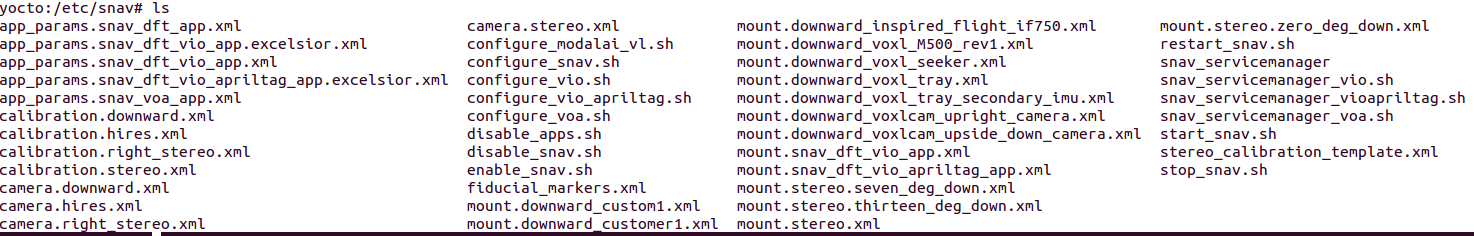• For the camera matrix i've checked in the ~/.ros/root/camera_info folder and they also non change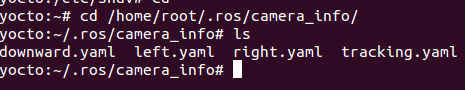• Ok, they should be exact. Did you remove vibration by adding foam?

• Yes, after adding some foam, The UAV now are stable than a bit. Do you think the battery should be problem? Our 3S battery are quite old.